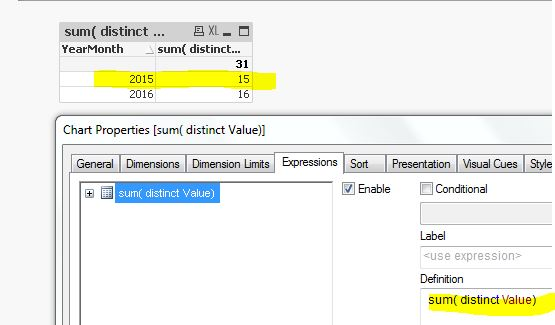New to Qlik Sense

Discussion board where members can get started with Qlik Sense.

Highlighted
New Contributor II

How to add 3 conditions in one expression in qlik sense.

Hi,

I have a dataset like below:

 Policy Number Type TOTAL 118 1 90 181 1 100 181 1 100 182 1 15 183 1 15 184 1 25 186 1 80 188 1 150 189 1 250

I have to include 3 below conditions in one expression.

1. Sum of field Total

2. Distinct of Policy Number

3. Type = 1

Like in above dataset, some policy number have same records with type 1 and so the total gets wrong so wanted to use all 3 below conditions.

I was trying with some what below but it din't work.

Sum( { <[TYPE] = {'1'},{<Distinct Policy_Number>}>} [TC_TOTAL]  )

Kindly help me to get it as it is required for my project.

9 RepliesPartner

Re: How to add 3 conditions in one expression in qlik sense.

maybe this:

sum( aggr( sum( {< TYPE = {'1'} >} [TC_TOTAL] ), Policy_Number ) )

Contributor II

Re: How to add 3 conditions in one expression in qlik sense.

I think you should Load Distinct the data set on Script Load

Something like this : Load Distinct * from abc ....

Then Chart Function :

sum({<[TYPE] = {'1'}>} Total)

Hope this helps.

Justin.

Honored Contributor II

Re: How to add 3 conditions in one expression in qlik sense.

Hi,

by default qlik will remove the duplicate. what is your expected output. Thanks

try below method to show the total amount by using set analysis

=sum( total  {\$<Type={1}>}TC_TOTAL)

or u can try this

=sum( {\$<Type={1},[Policy Number]={"=Count(distinct [Policy Number])"}>}TC_TOTAL)

Thanks,

Deva

Contributor II

Re: How to add 3 conditions in one expression in qlik sense.

Hi devarasu07,

I has test the Remove Duplicate

If you view on Table, the data duplicate not View (Only View unique)

But if you use Sum(Value), it's duplicates of sum

Examples : I have a duplicate data (2015 15)

LOAD * INLINE [

YearMonth, Value

2015, 15

2015, 15

2016, 16

];

Then I create Table :

Dimension : YearMonth  ,Value

Measure : Sum(Value)So I think we should Load Distinct *  from Table to Remove the duplicates data.

Regards,

Justin.

Honored Contributor II

Re: How to add 3 conditions in one expression in qlik sense.

Hi,

in that case, yes u can simple do the distinct function during the load script itself.

like this

Fact:

LOAD distinct * INLINE [

YearMonth, Value

2015, 15

2015, 15

2016, 16

];

Contributor II

Re: How to add 3 conditions in one expression in qlik sense.

Hi devarasu07,

I'm confuse "default qlik will remove the duplicate"

So I recommend Load Distinct the data, if you NOT load distinct and you use =sum( total  {\$<Type={1}>}TC_TOTAL)

I think the result will wrong (duplicates data not Remove)

I hope you understand meThank youJustin.

Honored Contributor II

Re: How to add 3 conditions in one expression in qlik sense.

Hi,

if you don't use distinct function in the load script then result will be aggregated by YearMonth dimension

like our sql query:  select YearMonth, sum(value) from fact group by YearMonth

note: if you just add your YearMonth field in a list box it will show only distinct value

but you case u don't want show the aggregated value so we simply use distinct keyword to remove the duplicated row and it will return only the unique record.

LOAD distinct * INLINE [

YearMonth, Value

2015, 15

2015, 15   ---> this entire row will be removed

2016, 16

];

or by using set analysis also u can remove that duplicate row

sum( distinct Value)

or

=sum( distinct  total  {\$<Type={1}>}TC_TOTAL)also check this article about the aggregation and distinct function,

https://www.analyticsvidhya.com/blog/2014/02/aggr/

QlikView App: Aggr()

http://www.qlikfix.com/2013/07/30/distinct-can-be-deceiving/

Thanks,
Deva

Contributor II

Re: How to add 3 conditions in one expression in qlik sense.

It's greats!

Thank youJustin.

Honored Contributor II

Re: How to add 3 conditions in one expression in qlik sense.

Hi,

Once your clear, you can proceed to close this thread by marking helpful and correct. it might useful for others. Thanks Deva